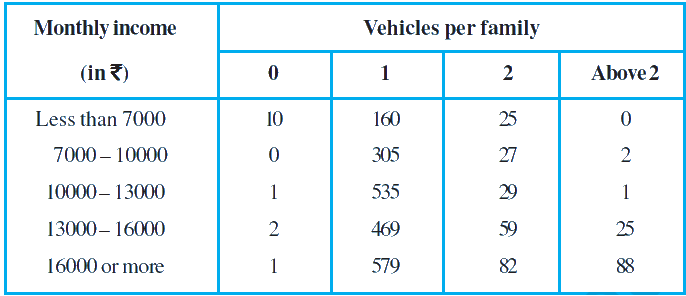In the verge of coronavirus pandemic, we are providing FREE access to our entire Online Curriculum to ensure Learning Doesn't STOP!

# Ex.15.1 Q5 Probability Solution - NCERT Maths Class 9

Go back to  'Ex.15.1'

## Question

An organization selected $$2400$$ families at random and surveyed them to determine a relationship between income level and the number of vehicles in a family. The information gathered is listed in the table below.Suppose a family is chosen. Find the probability that the family chosen is

(i) earning $$\rm Rs 10000 – 13000$$ per month and owning exactly $$2$$ vehicles.

(ii) earning $$\rm Rs 16000$$ or more per month and owning exactly $$1$$ vehicle.

(iii) earning less than $$\rm Rs 7000$$ per month and does not own any vehicle.

(iv) earning $$\rm Rs 13000 – 16000$$ per month and owning more than $$2$$ vehicles.

(v) owning not more than $$1$$ vehicle.

Video Solution
Probability
Ex exercise-15-1 | Question 5

## Text Solution

What is known?

Family monthly income and vehicles per family.

What is unknown?

Probability of family owning $$0$$ or $$1$$ or $$2$$ or above $$2$$ vehicles based on the incomes.

Reasoning:

The empirical probability $$P(E)$$ of an event $$E$$ happening, is given by:

\begin{align} & { = \frac{ {\text{Number of times }} {\text{heads occur}} }{{{\text{Total number of tosses}}}}} \\ &=\frac{72}{200} \\ &=\frac{9}{25} \end{align}

Use probability to derive the solution where

Probability (of family owning vehicle based on the earnings)

\begin{align} = \frac{ \begin{bmatrix} \text{No of vehicles owned} \\ \text{ based on earnings} \end{bmatrix} }{ \text{Total number} \\ \text{ of families} } \end{align}

Steps:

Total no of families $$= 2400$$

(i)

Probability $$P1$$ (a family earning $$\rm Rs 13000$$ to $$\rm Rs 10000$$ per month and owning exactly $$2$$ vehicles)

\begin{align} &=\!\frac{ \begin{bmatrix} \text{No of a families earning Rs 13000}\\\text{to Rs 10000 per month and} \\ \text{owning exactly 2 vehicles} \end{bmatrix} }{{{\text{Total number of families}}}}\\& = \,\frac{{{\rm{29}}}}{{{\rm{2400}}}}\end{align}

(ii)

Probability $$P2$$ (a family earning $$\rm Rs 16000$$ per month and owning exactly $$1$$ vehicle)

\begin{align} &=\frac{ \begin{bmatrix} \text{No of a families earning}\\\text{ Rs 16000 per month and }\\\text{owning exactly 1 vehicle} \end{bmatrix} }{{{\text{Total number of families}}}}\\ \\ &= \frac{{{\rm{579}}}}{{{\rm{2400}}}}\, = \frac{{193}}{{800}}\end{align}

(iii)

Probability $$P3$$ (a family earning less than $$\rm Rs 7000$$ per month and does not own any vehicle)

\begin{align}& =\! \frac{\begin{bmatrix} \text{No of a families earning} \\\text{less than Rs 7000 per month} \\ \text{and does not own any vehicle} \end{bmatrix} }{{{\text{Total number of families}}}}\\ \\ &= \,\frac{{10}}{{{\rm{2400}}}}\\&= \frac{1}{{240}}\end{align}

(iv)

Probability $$P4$$ (a family earning Rs $$13000$$ to Rs $$16000$$ per month and owning more than $$2$$ vehicles)

\begin{align} &= \frac{ \begin{bmatrix} \text{No of a families earning } \\ \text{Rs 16000 to Rs 13000 per } \\ \text{month and owning more }\\\text{ than 2 vehicles}\end{bmatrix} }{{{\text{Total number of families}}}}\\ & = \,\frac{{{\rm{25}}}}{{{\rm{2400}}}} = \frac{1}{{96}}\end{align}

(v)

Probability $$P5$$ (a family owning $$0$$ or $$1$$ vehicle)

Total number of families

\begin{align} & = \,\frac{\begin{bmatrix} 10 + 0 + 1 + 2 + 1 + 160 \\ + 305 + 535 + 469 + 579 \end{bmatrix} }{{{\rm{2400}}}} \\ \\ &= \frac{{2062}}{{2400}} = \frac{{1031}}{{1200}}\end{align}

Learn from the best math teachers and top your exams

• Live one on one classroom and doubt clearing
• Practice worksheets in and after class for conceptual clarity
• Personalized curriculum to keep up with school